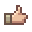# PCCollecting All Dev Sets; Check My Math?

#### tallstar27

##### Skeletron Prime
Just so you're warned, there's gonna be a lot of math here.

So, I want to get all the dev armor sets, and I was wondering what the probability of that happening was, so I could plan how many summon items to acquire. The results actually scared me a lot so I was hoping I did something wrong and someone could help me figure this out.

To start: there are 14 dev armor sets (http://terraria.wiki.gg/Developer_items; Cenx has 2 separate sets) that drop at 0.5% or 1/20 from each treasure bag. That means the odds of getting a new dev set is (1/20)*([14-n]/14), where n is the number of sets you already have.

I'll present the formula I came up with and how I simplified it.

{1/20}*(14/14)*{1/20}*(13/14)*{1/20}*(12/14)*...{1/20}*(1/14)

({1/20}^14)*[(14/14)*(13/14)*(12/14)*...(1/14)]

({1/20}^14)*((1/14)^14)*[14*13*12*...1]

({1/20}^14)*((1/14)^14)*14!

This equation yields 4.78846e-24, or 0.00000000000000000000000478846 (that's 23 zeroes after the decimal point), which as far as I can tell (no decimal to fraction site I could find would handle such a small number) is roughly 1 in 250,000,000,000,000,000,000,000.

Someone please tell me I did something wrong, because I don't really want to fight 250 sextillion Duke Fishron.#### Orion_Bright

##### Retinazer
Just so you're warned, there's gonna be a lot of math here.

So, I want to get all the dev armor sets, and I was wondering what the probability of that happening was, so I could plan how many summon items to acquire. The results actually scared me a lot so I was hoping I did something wrong and someone could help me figure this out.

To start: there are 14 dev armor sets (http://terraria.wiki.gg/Developer_items; Cenx has 2 separate sets) that drop at 0.5% or 1/20 from each treasure bag. That means the odds of getting a new dev set is (1/20)*([14-n]/14), where n is the number of sets you already have.

I'll present the formula I came up with and how I simplified it.

{1/20}*(14/14)*{1/20}*(13/14)*{1/20}*(12/14)*...{1/20}*(1/14)

({1/20}^14)*[(14/14)*(13/14)*(12/14)*...(1/14)]

({1/20}^14)*((1/14)^14)*[14*13*12*...1]

({1/20}^14)*((1/14)^14)*14!

This equation yields 4.78846e-24, or 0.00000000000000000000000478846 (that's 23 zeroes after the decimal point), which as far as I can tell (no decimal to fraction site I could find would handle such a small number) is roughly 1 in 250,000,000,000,000,000,000,000.

Someone please tell me I did something wrong, because I don't really want to fight 250 sextillion Duke Fishron.You could just grind EoC. It would still drop the sets in its bags couldn't it?

#### KM132

##### Spazmatism
The problem here seems to be that you've multiplied all the chances together; the probably you came up with is the probability of getting every dev armor set back-to-back (14 treasure bags, 14 unique sets)

If you were to find out about how many summons you'd need, you should add the chances together. The equation I came up with is, which results in about 910 treasure bags to get every last dev set. It's still a daunting task, but it's a bit more possible than hoping for a 1-in-250 sextillion chance.

•tallstar27

#### tallstar27

##### Skeletron Prime
You could just grind EoC. It would still drop the sets in its bags couldn't it?
Unfortunately not; the sets only drop from hard mode bosses' bags, and even then that would be a LOT of lenses.
The problem here seems to be that you've multiplied all the chances together; the probably you came up with is the probability of getting every dev armor set back-to-back (14 treasure bags, 14 unique sets)

If you were to find out about how many summons you'd need, you should add the chances together. The equation I came up with is, which results in about 910 treasure bags to get every last dev set. It's still a daunting task, but it's a bit more possible than hoping for a 1-in-250 sextillion chance.
That makes a lot of sense actually! I would have thought for sure they were multiplied... I was under the impression that when you wanted something "and" something you multiplied them the probabilities and if you wanted something "or" something you added them, and since I wanted this set AND that set and so forth I would be multiplying-but your answer makes a lot more sense! It reflects what's been happening as I've farmed bosses as well. Thanks so much!